AdfTest is a test for unit root in a time series sample. But, the rejection of the null hypothesis is often considered as an evidence of stationarity. The augmented Dickey–Fuller (ADF) statistic, used in the test, is a negative number. The more negative it is, the stronger the rejection of the hypothesis that there is a unit roots at some level of confidence. AdfTest indicator returns 4 values:

• CValue - the c-value of the test statistic

• Decision - the decision for the test. 1.0 if the null hypothesis is accepted (series has unit root); 0.0 if the null hypothesis is rejected (series is stationary)

• PValue - the p-value of the test statistic

• Statistic - the statistic of AdfTestCalculation

The Dickey-Fuller model is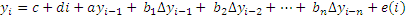where L is the lag operator: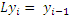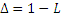, so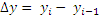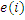is the innovations process.

The null hypothesis restricts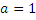.

The test assesses the null hypothesis under the model variant appropriate for series with different growth characteristics (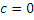or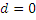). Lagged differences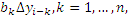"augment" the test to account for serial correlations in the innovations process.Chart Example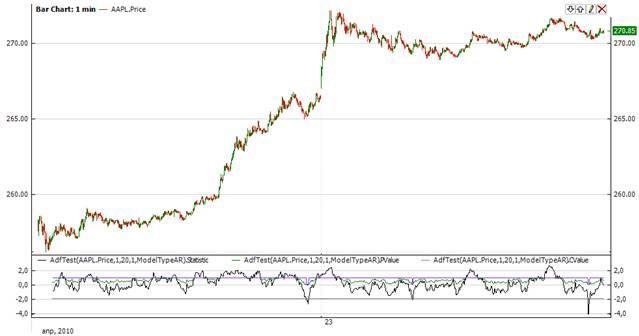Implementation and Usage

To initialize AdfTest indicator use one of the constructors provided:

AdfTest – this constructor sets default value 1 for update period, 20 for working period and ModelTypeAR for the type of time series model.

AdfTest(Int32, Int32, TimeSeriesModelType) – creates ADF Test indicator with expanding window and update scheduled by point period

AdfTest(TimeSpan, Int32, TimeSeriesModelType) – creates ADF Test indicator with expanding window and update scheduled by point period. updatePeriod parameter is of TimeSpan type.

To operate with sliding data sets, it is necessary to define the sliding window size value as a time period or a number of data points in the following constructors (note that updatePeriod can take either TimeSpan or int value):

AdfTest(Int32, Int32, Int32, TimeSeriesModelType) - initializes an instance of AdfTest class with sliding window and update sheduled by point period.

AdfTest(Int32, TimeSpan, Int32, TimeSeriesModelType) - initializes an instance of AdfTest class with sliding window and update sheduled by time period.

AdfTest(Int32, TimeSpan, Int32, TimeSeriesModelType) - initializes an instance of AdfTest class with sliding window and update sheduled by point period. workingPeriod is of TimeSpan type.

AdfTest(TimeSpan, TimeSpan, Int32, TimeSeriesModelType) - initializes an instance of AdfTest class with sliding window and update sheduled by time period. workingPeriod is of TimeSpan type.

Use

CValue

PValue

Decision

Statistic

properties to get current value.Example
C#
``` 1// Create new instance
3
4// Number of stored values
6
9
10// Get indicator values